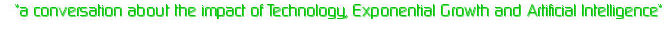# Standard growth model

by Marvin
(Fargo, ND USA)

The standard growth model I learned in Differential equations was for a given population

dp/dt=a(1)p+a(2)p*p+a(3)p^3+a(n)p^n
Usually the first two terms dominate so completely we may model the rate of growth as

dp/dt= ap-bp^2 where a and b are positive constants.
The ultimate (teminal population)
0=ap-bp*p -> ap=bp*p -> a=bp p=a/b

inflection point = a/2b at half of the final value

Note until; the inflection point is reached the differential equation when plotted seems to be exponential.

In human system the usual time (since the industrial revolution) for a technology to reach the ultimate population is about 100 years.

The ultimate population is twice the population at the inflection point of the graph usually reached at 50 years (two human generations). The inflection point occurs when limits of a given technology have been reached and what remains is the market filling every possible niche.

Examples of technological developmentused are piston steam engines 1774 to 1874 by 1834 the 440 steam engine had been built and dominated rairoading for the next 50 years improvements were technically minor as the multi expansion engine had already been in use by thei time.
Internal combution engines 1865 to 1965 (advance ssince 1965 have not been engines, but in numerical control systems.

Aircraft 1903 to 2003

From the Wright bros to supersonic transport.

So in conclusion moore's law should be "ending" about now as the two generations have passed.